Got Feedback?

Found a bug? Have a suggestion? Fill the form below and we'll take a look!

X

Quadratic calculator is the very useful as it shows each and every steps very precisely. Whenever any quadratic equation consider to solve for unknown, we just enter the co-efficient of x2, x and the constant and within second it will share the output of entered input values. Basically quadratic calculator is a free online tool that shows the discriminant and provides roots of the given quadratic equation it gives accurate and quick result. Whenever we wish either graph or roots.
In short, we can say that quadratic calculator has capacity to solve quadratic equation using quadratic equation. Whenever the question comes in our mind how to find out how many roots does quadratic equation has?
Quadratic calculator is the best option in this context.

$$a x^2 + bx + c = 0$$

$$x = \dfrac{ -b \pm \sqrt{△}}{ 2a }$$

The quadratic equation has only one root when $$△ = 0$$. The solution is equal to $$x = \frac{-b}{2a}$$ it is called repeated or double roots.

The quadratic equation has no real solution for $$△ < 0$$.

Notice that as $$△ < 0$$, the square root of the determinant will be an imaginary value

Hence,

$$Re(x) = \dfrac{-b}{2a}$$

$$lm(x) = \pm \dfrac{△}{2a}$$

There are three main forms of quadratic equations:
1- Standard form
2- Factored form
3- Vertex form

## Roots Of The Quadratic Equation

The values of x satisfying the equations are called the roots of the equation. A second degree equation which contain at least one term that is Squared.

## Methods to Solve Quadratic Equations

Quadratic equation can be solved in multiple ways
1- Factoring
3- Completing the square
4- Graphing

## How to drive Quadratic Formula

In general, the steps taken to solve a quadratic equation $$x^2 + bx + c = 0$$ where a, b and c are real numbers, by completing the square.

$$ax^2 + bx + c = 0$$

Divide throughout by “a”:

$$\dfrac{a}{a}x^2 + \dfrac{b}{a}x + \dfrac{c}{a} = 0$$

$$x^2 + \dfrac{b}{a}x + \dfrac{c}{a} = 0$$

Rewrite the equation such that the constant term is on the RHS of the equation:

$$x^2 + \dfrac{b}{a}x = -\dfrac{c}{a}$$

Add $$(\frac{b}{2a})^2$$ to both sides of the equation to make LHS a perfect square:

$$x^2 + \dfrac{b}{a}x + (\dfrac{b}{2a})^2 = -\dfrac{c}{a} + (\dfrac{b}{2a})^2$$

Factorise the expression on the LHS and simplify RHS:

$$(x + \dfrac{b}{2a})^2 = -\dfrac{c}{a} + \dfrac{b^2}{4a^2}$$

$$(x + \dfrac{b}{2a})^2 = \dfrac{b^2 – 4ac}{4a^2}$$

Take sqaure root on both sides

$$\sqrt{(x + \dfrac{b}{2a})^2} = \pm \sqrt{\dfrac{b^2 – 4ac}{4a^2}}$$

$$x + \dfrac{b}{2a} = \pm \dfrac{\sqrt{b^2 – 4ac}}{2a}$$

$$x = -\dfrac{b}{2a} \pm \dfrac{\sqrt{b^2 – 4ac}}{2a}$$

$$x = \dfrac{-b \pm \sqrt{b^2 – 4ac}}{2a}$$

## Graphing

The X value found through the quadratic formula are roots of quadratic equation. X values where any parabola cross the x-axis.

Quadratic formula also define the axis of symmetry of the parabola.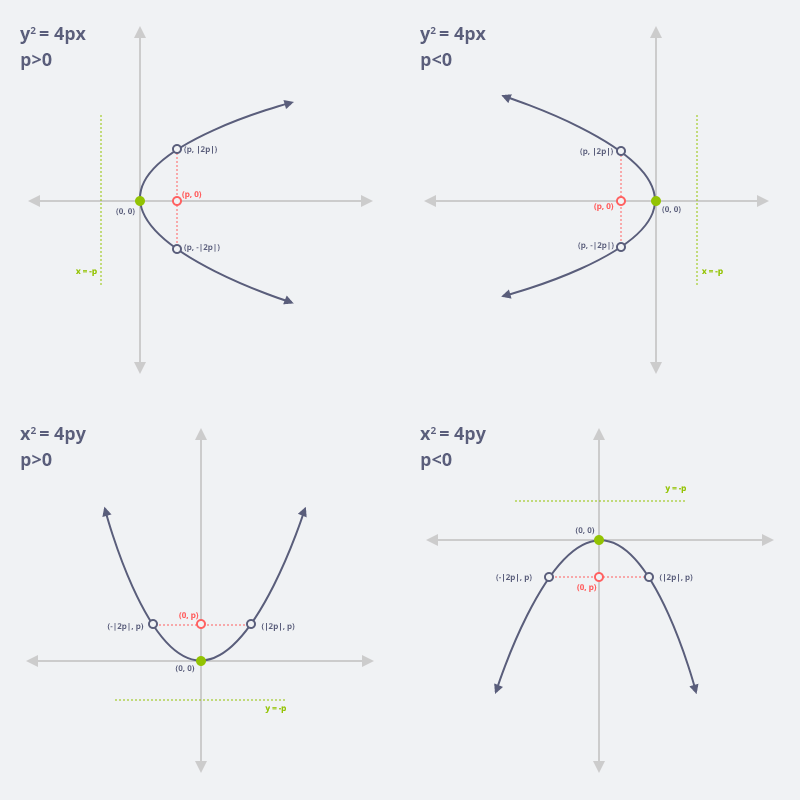Our quadratic equation solver will solve a second degree polynomial equation such as $$ax^2 + bx + c = 0$$ for x, where $$a ≠ 0$$ for real and complex root where x is an unknown, A is referred to as the quadratic co-efficient, B is the linear co-efficient and C Is the constant. By considering discriminant value $$b^2 – 4ac$$, we can assess the nature of the roots.
Check our Discriminant Calculator.

When
$$b^2 – 4ac < 0$$ there is no real root.
$$b^2 – 4ac = 0$$ there is one real root.
$$b^2 – 4ac < 0$$ there is no real root.

Note: Quadratic equation are commonly used in situations where two things are multiplied together and they both depend on the same variable. For example, when working with area, if both dimensions are written in terms of the same variable we use a quadratic equation.

When we solve quadratic equation by graphing method, here x values are found through the quadratic formula where any parabola crosses the x-axis. Quadratic formula also define the axis of symmetry of the parabola. If any equation that can be rewrite in $$ax^2 + bx + c = 0$$ form can be solved by using quadratic formula.

We can also graph the function $$y = ax^2 + bx + c$$. Its shape is parabola and the roots of the quadratic equation are the x-intercepts of this function.

## A Random Image Of Cup-Up Parabola

$$y = 2x^2 – 4x – 1$$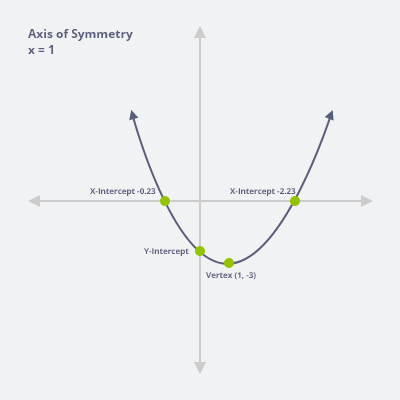## A Random Image Of Cup-Down Parabola

$$x^2 – 2x + 1 = 0$$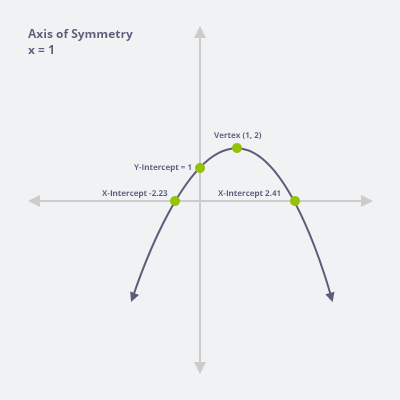For more details visit Graph Presentation “Parabola” Of A Quadratic Equation

Note: The above mentioned formula for solving quadratic equation is normally used when the quadratic expression cannot be factorized easily.

Note: Another method that can be used to find the solutions of quadratic equation $$ax^2 + bx + c = 0$$ is by drawing the corresponding quadratic graph of $$y = ax^2 + bx + c$$ and to find the x–coordinates of the points of intercepts of the graphs with the x-axis (y = 0).

Regardless of which method you are adopting you first need to check whether the quadratic equation should equal to zero.

## Examples

### Solve the equation $$3x^2 + 4x – 5 = 0$$

Solution: It is a quadratic equation but it can be solved by factorization so we should opt for quadratic formula or either completing square method first.
For more details visit Quadratic Equation by Factoring Method

By completing the square method

$$3x^2 + 4x – 5 = 0$$

$$3x^2 + 4x = 5$$

$$x^2 + \dfrac{4}{3}x = \dfrac{5}{3}$$

$$x^2 + 2(\dfrac{2}{3})(x) + (\dfrac{2}{3})^2 = \dfrac{5}{3} + (\dfrac{2}{3})^2$$

$$( x + \dfrac{2}{3})^2 = \dfrac{5}{3} + \dfrac{4}{9}$$

$$x + \dfrac{2}{3} = \pm \dfrac{\sqrt{19}}{9}$$

$$x = -2.12$$ and $$x = 0.78$$ are the roots of the equation

Comparing $$3x^2 + 4x – 5 = 0$$ which $$ax^2 + bx + c = 0$$

We have $$a = 3$$, $$b = 4$$ and $$c = 5$$

After substituting the above values in quadratic formula,

$$x = \dfrac{ -b \pm \sqrt{b^2 – 4ac}}{ 2a }$$

Result: $$x = 0.786$$ or $$x = -2.12$$

### Solve the equation $$y = x^2 – 4x + 4$$

By graphical method

Complete the table

 x y -1 0 1 2 3 4 5

Draw the graph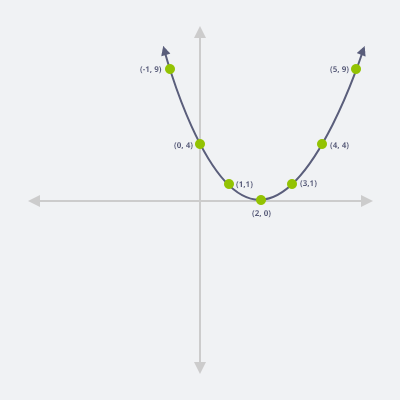Now table will be

 x y -1 0 1 2 3 4 5 9 4 1 0 1 4 9

From the graph, the curve $$y = x^2 – 4x + 4$$ touches the x-axis at $$x = 2$$ only. Therefore, the solution of the equation $$y = x^2 – 4x + 4$$ is $$x = 2$$.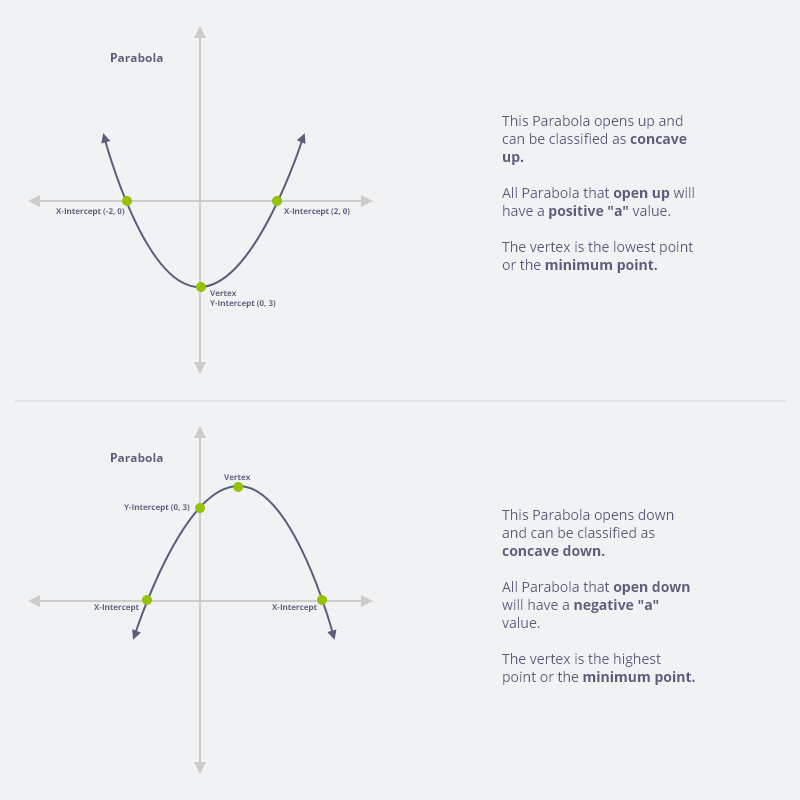## Quadratic Functions And Their Graphs: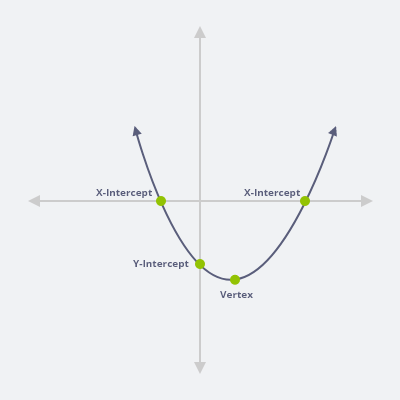## Standard form of Quadratic Equation

$$ax^2 + bx + c = 0$$

This is the quadratic equation in standard form. In this form of quadratic equations we have,

• a, b and c are constants
• a is not equals to zero ($$a ≠ 0$$)

### For Example:

Lets suppose three equations A, B and C.

Equation A: $$x^2 + 5x + 2 = 0$$, here $$a = 1$$, $$b = 5$$ and $$c = 2$$

Equation B: $$3x^2 + x + 9 = 0$$, here $$a = 3$$, $$b = 1$$ and $$c = 9$$

Equation C: $$x + 9 = 0$$, here $$a = 0$$, $$b = 1$$ and $$c = 9$$

Equation A and B are standard form of quadratic equation, but equation C not belongs to standard form of quadratic because in this equation, $$a = 0$$

## Pure/Incomplete Form Of A Quadratic Equation

Any equation of the form $$ax^2 + bx + c = 0$$, in the variable x, here a, b, c are real numbers and $$a ≠ 0$$ or the quadratic equation having only second degree variable is called a pure quadratic equation.

$$ax^2 + bx + c = 0$$ is called incomplete. In which, either b or c or both equal to 0. Such equation can be easily solved by applying basic algebraic rule. For example, $$x^2 – 49 = 0$$. Thus, to solve such equation we need to take square root on both the sides so $$x^2 = 49$$ becomes $$x = \pm 7$$. So, a quadratic equation that does not contain a first power variable (x term) is called an incomplete or pure quadratic equation.

## Hidden Form Of A Quadratic Equation

Often we encounter/came across an equation that is a quadratic in disguise. For example, $$x^4 + 5x^2 – 6 = 0$$

It’s a fourth degree equation it means exponent of the leading term is twice the second term. Here we have to tackle it by substitution

Let $$z = x^2$$

Then, $$x^4 = z^2$$

Then the equation in terms of z becomes:

$$z^2 + 5z – 6 = 0$$

Now, it clear reflect quadratic equation form.

Hence, the roots are $$z = -3$$ and $$z = 1$$.

Since $$z = x^2$$ we have that $$x^2 = 1$$ or $$x^2 = -3$$

The first has solution $$x = 1$$ or $$x = -1$$

And the second has no solution.

## FAQS

Q1: How to identify the quadratic equation?

Answer: Equation can be easily identified if it has highest power is two we can define quadratic equation as an equation of degree 2, which means that the highest exponent or power of this function is two. The standard form of a quadratic equation is $$y = ax^2+ bx + c$$.

Q2: How can we apply a quadratic equation in our daily life problem solving?

Answer: There are a number of application, few of them are design of bridges, space science, satellite dishes that is designed to receive and transmit information by radio waves also has a parabolic types of shapes. Parabola can be seen in nature or in manner you can say that from the path of the throwing a baseball to the satellite dishes to fountains.

Q3: How can we draw the graph of quadratic equation?

Answer: We can draw the graph of quadratic equation in two variables
2- Determine whether the parabola opens upward or downward.
3- Find the axis of symmetry.
4- Find the vertex.
5- Find x and y-intercepts.
6- Graph the parabola.

Q4: How to find the co-ordinates of vertices?

1- Get the equation in the form of $$y = ax^2 + bx + c$$.
2- Calculate $$\frac{–b}{2a}$$. This is the x-coordinate of the vertex.
3- To find the y co-ordinate of the vertex, simply plug the value of $$\frac{–b}{2a}$$ into the equation for x and solve for y.

Q5: How to find intercepts of the equation of the quadratic function?

Answer: Let an example to find x and y intercepts of the quadratic function $$y = 2x^2- 4x – 1$$
For y-intercept: $$x = 0$$ => $$y = 2(0)^2 – 4(0) – 1$$ => y-intercept: (0, -1)
For x-intercept: $$y = 0$$ => $$0 = 2x^2 – 4x – 1$$, after applying quadratic formula x-intercept: ( $$1 \pm \sqrt{\frac{3}{2}}$$, 0 )

Q6: How to write an equation of the axis of symmetry?

Answer: The x-coordinate of the vertex is the equation of the axis of symmetry of the parabola. For a quadratic function in standard form, $$y = ax^2 + bx + c$$, the axis of symmetry is a vertical line that divides the parabola $$x = -\frac{b}{2a}$$.

Q7: How to draw/sketch the graph of the quadratic function?

Answer: A quadratic equation is drawn as a curve on a set of axes. This type of curve is called a parabola and it is a symmetrical number.
1- To draw the graph, we need coordinates.
2- We generate these coordinates by substituting values in the quadratic equation.

Q8: Is it possible to solve a quadratic equation if, it does not factorise?

Answer: Yes, it is possible, either considering completing square method or applying quadratic formula.

Q9: Why is it called the quadratic?

Answer: In mathematics, a quadratic is a type of problem that deals with a variable multiplied by itself an operation known as a squaring.

Q10: What are the four ways to solve a quadratic equation?

Answer: There are basically 4 ways:
1- By factorisation.
2- By completing square method.
3- By graphical method.